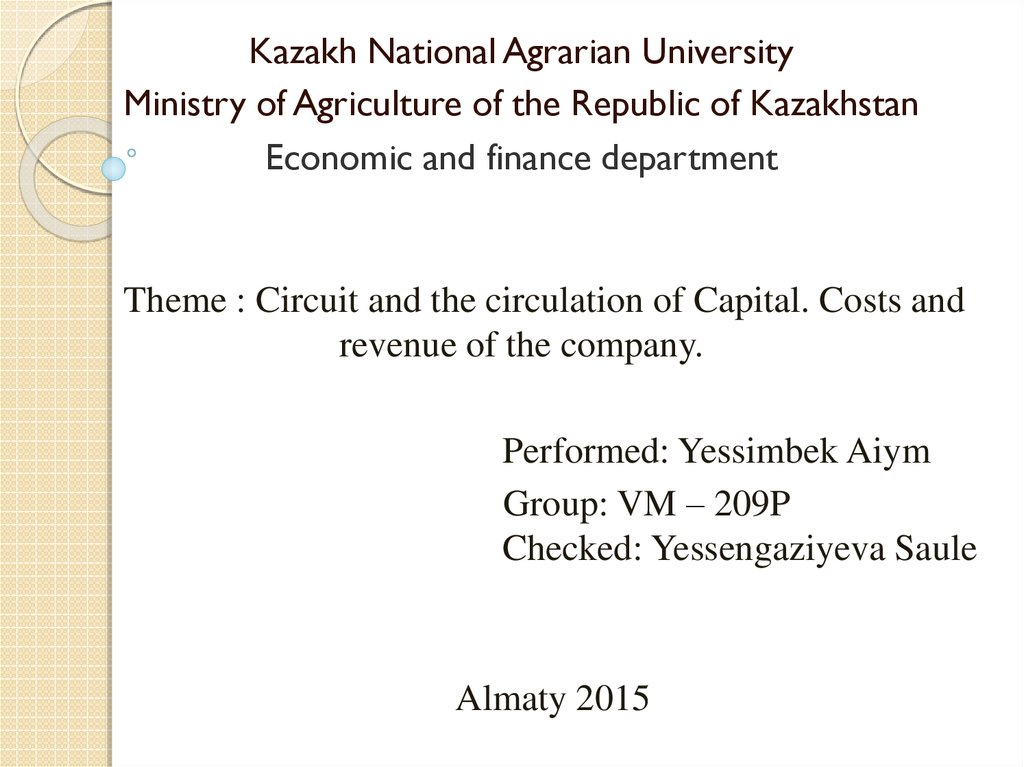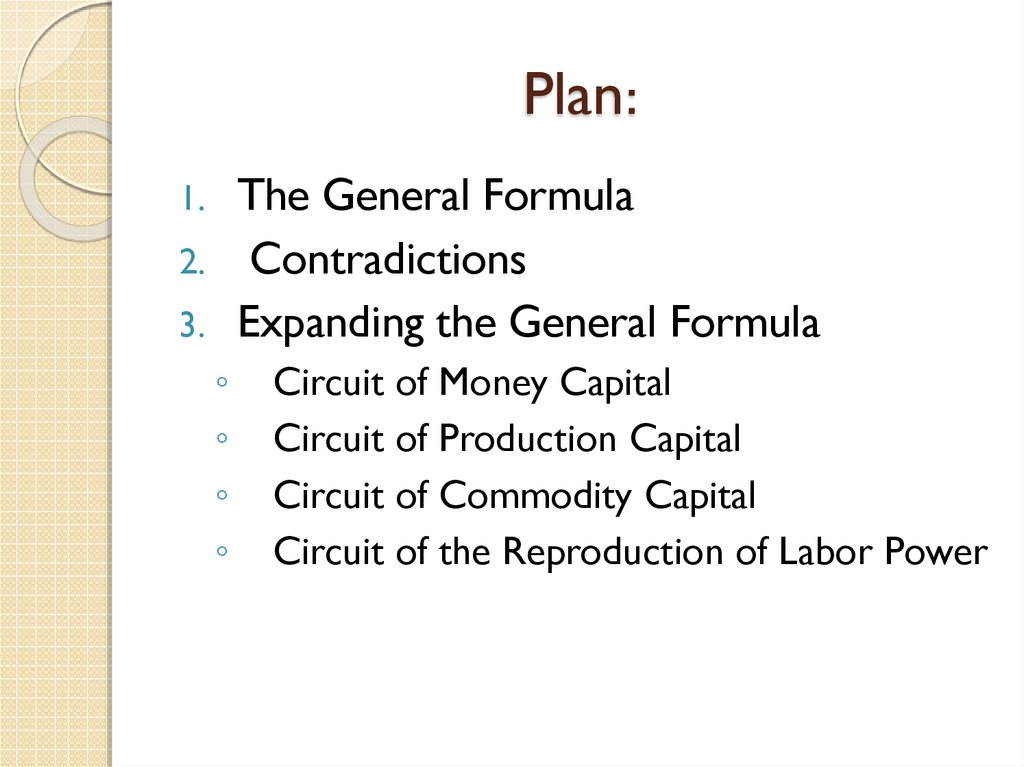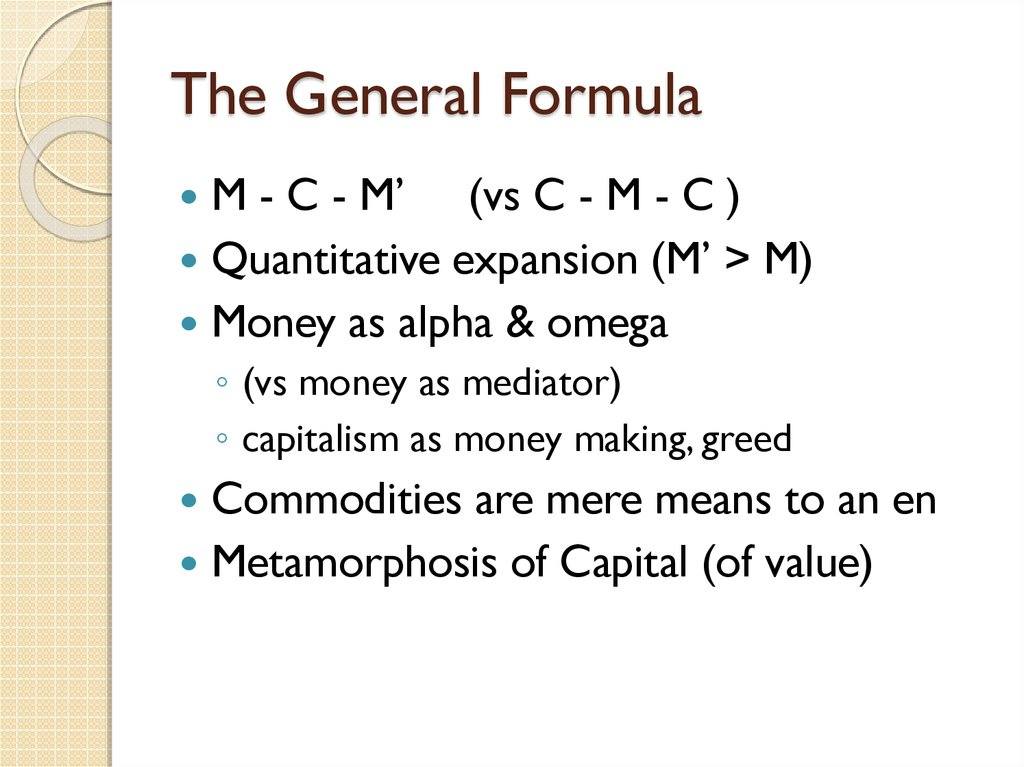Circuit and the circulation of Capital. Costs and revenue of the company

1.

Kazakh National Agrarian University
Ministry of Agriculture of the Republic of Kazakhstan
Economic and finance department
Theme : Circuit and the circulation of Capital. Costs and
revenue of the company.
Performed: Yessimbek Aiym
Group: VM – 209P
Checked: Yessengaziyeva Saule
Almaty 2015

2. Plan:

The General Formula
Expanding the General Formula
1.
2.
3.

Circuit of Money Capital
Circuit of Production Capital
Circuit of Commodity Capital
Circuit of the Reproduction of Labor Power

3. The General Formula

M - C - M’ (vs C - M - C )
Quantitative expansion (M’ > M)
Money as alpha & omega
◦ (vs money as mediator)
◦ capitalism as money making, greed
Commodities are mere means to an en
Metamorphosis of Capital (of value)

Problem: Explain source of “prime” in M’
Marx assumes equal exchange
So M’-M cannot originate in exchange
Unequal exchange, e.g., cheating, would
merely redistribute value
Ques: If not in circulation, then where?
Ans: In production!

5. Circuit of Money Capital -1

M - C - M’ becomes:
M - C(LP, MP) . . . P . . . C’ - M’, where
M - C = factor market exchanges
LP = labor power
MP = means of production
...P... = production process
C’ = produced commodity with expanded value
C’ - M’ = sale of commodity

6. Circuit of Money Capital –2

LP & M - C(LP) are discussed in Chap 6
...P... is discussed in Chap 7
NB: Money, as one moment of circuit, is
reproduced in the circuit
M as money to be invested is reproduced as M’ as
profit
BUT: M - C - M’ is endlessly repeated as
M - C - M’.M’ - C” - M’’.M’’ - C” - M”’ etc

M”
M’
endless
expansion
C”’
C”
M
C
C’
...P...
...P...
...P...
C’
C”

8. Circuit of Production Capital

Circuit of Money Capital
M - C(LP,MP) ...P... C’ - M’.M - C (LP,MP) ...P...
Capital
Circuit of Productive
Reproduction of relations of production, LP &
MP, within the overall circuit
Closest to “socio-political” point of view, focus
on work

9. An Absence?

Circuit of Money Capital
Productive Capital
Circuit of
M - C(LP,MP) ...P... C’ - M’.M’ - C
(LP,MP)...P...C”
Circuit of Commodity
Capital

10.

Circuit of Money Capital
Circuit of Productive Capital
M - C(LP,MP) ...P... C’ - M’.M’ - C (LP,MP)...P...C”
Circuit of Commodity Capital
What is left out?
Question: The reproduction of which aspect of the circuit
is left unaccounted for?

11. Circuit of Reproduction of Labor Power-1

Where is labor power reproduced?
To some degree within the circuit,
workers maintain their skills and abilities
by using them.
To a greater degree outside the circuit, in
“consumption,” daily life, the family.
The wage buys the means of
“reproduction”

12. Circuit of Reproduction of Labor Power-2

Marx: LP - M - C(MS)
◦ LP = labor power
◦ M = wage
◦ C(MS) = means of subsistence/reproduction
This makes Consumption/life goal of
circuit
◦ --appropriate from worker’s point of view
◦ --INappropriate from capital’s point of view

13. Circuit of Reproduction of Labor Power-3

Capital needs C(MS) to reproduce
worker
C(MS) must “produce” (...P...) new LP
So:
LP - M - C(MS) . . . P . . . LP*
◦ Where LP* = reproduced labor power
◦ LP* might be greater (kids, more skill)
◦ LP* might be smaller (less wage required)

14. Circuit of Reproduction of Labor Power-4

LP - M - C(MS) ...P... LP*. LP - M - C(MS)
M - LP
M - LP
. . . P . . . C’ - M’.
...P ..
.
M - MP
M - MP
Capitalist Circuit: Life reduced to reproduction
of labor power for capital, ready for labor
market

15. Reproduction of Labor Power?

This takes us to Chapter 6 which
discusses both the labor market and the
value of labor power.
We need to investigate:

LP - M
M - C(MS)
...P ...
LP* (why an asterik instead of a prime?)

17. References

1.
2.
3.
4.
5.
6.
7.
8.
Marx K. Kapital, vol. 2. In K. Marx and F. Engels, Soch.,
2nd ed., vol. 24.
V. M. RULEV
From Manuscript II. — F.E.
Beginning of Manuscript VII, started July 1878.
End of Manuscript VII. Beginning of Manuscript VI.
— F.E.
End of Manuscript VI. Beginning of Manuscript V. —
F.E.
This is true no matter how we separate capitalvalue and surplus-value. 10,000 lbs.
. Chuprov, Railroading, Moscow, 1875, pp. 69 and 70.